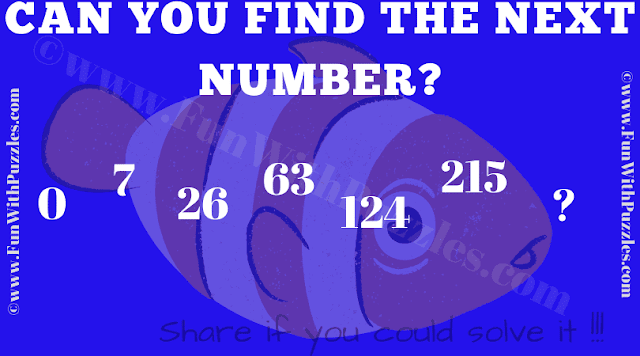This Genius Puzzle Series is part of Crack the Code Puzzle Game. In this puzzle first, you need to solve the given number series to get the next number in the series. It will be a 3-digit number which will be used as Code C in the final Crack the Code Puzzle Game. You also need to solve the other two puzzles titled Maths Logic Puzzle Question and Logical Reasoning Question to get the 3 digit codes named A and B respectively. Let's see if you can solve this 3 digit code and then solve the final Crack the Code Puzzle?C. Can you crack this puzzle to get the 3-digit code?
The answer to this "Genius Puzzle Series", is not provided. Solve this puzzle to get the 3 digit code and put in the final Crack the Code Puzzle Game as mentioned below in place of C.

Giša said...

342